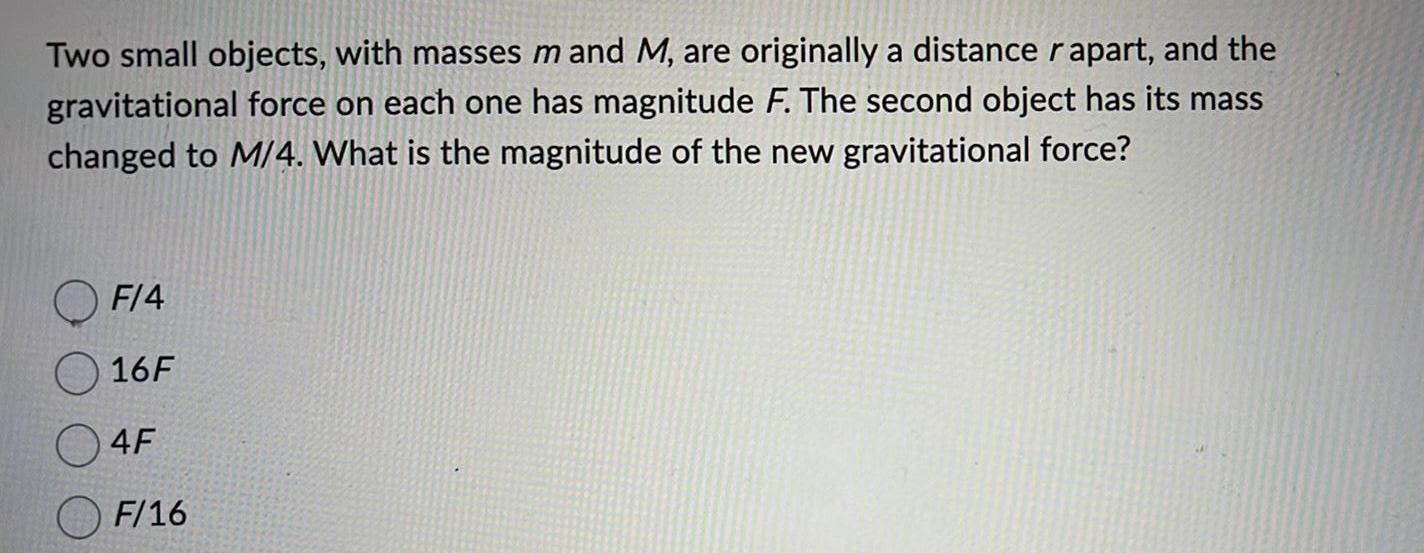Physics
Gravitation
Two small objects, with masses mand M, are originally a distance rapart, and the gravitational force on each one has magnitude F. The second object has its mass changed to M/4. What is the magnitude of the new gravitational force? F/4 16F 4F F/16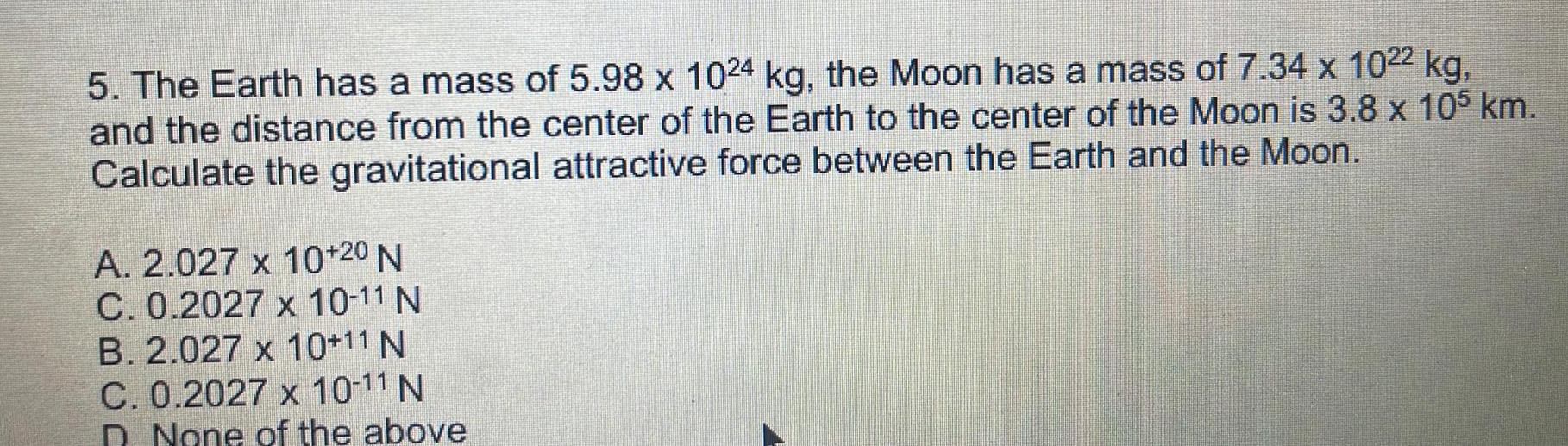Physics
Gravitation
The Earth has a mass of 5.98 x 1024 kg, the Moon has a mass of 7.34 x 1022 kg, and the distance from the center of the Earth to the center of the Moon is 3.8 x 105 km. Calculate the gravitational attractive force between the Earth and the Moon. A. 2.027 x 10^+20 N C. 0.2027 x 10^-11 N B. 2.027 x 10^+11 N C. 0.2027 x 10^-11 N D. None of the above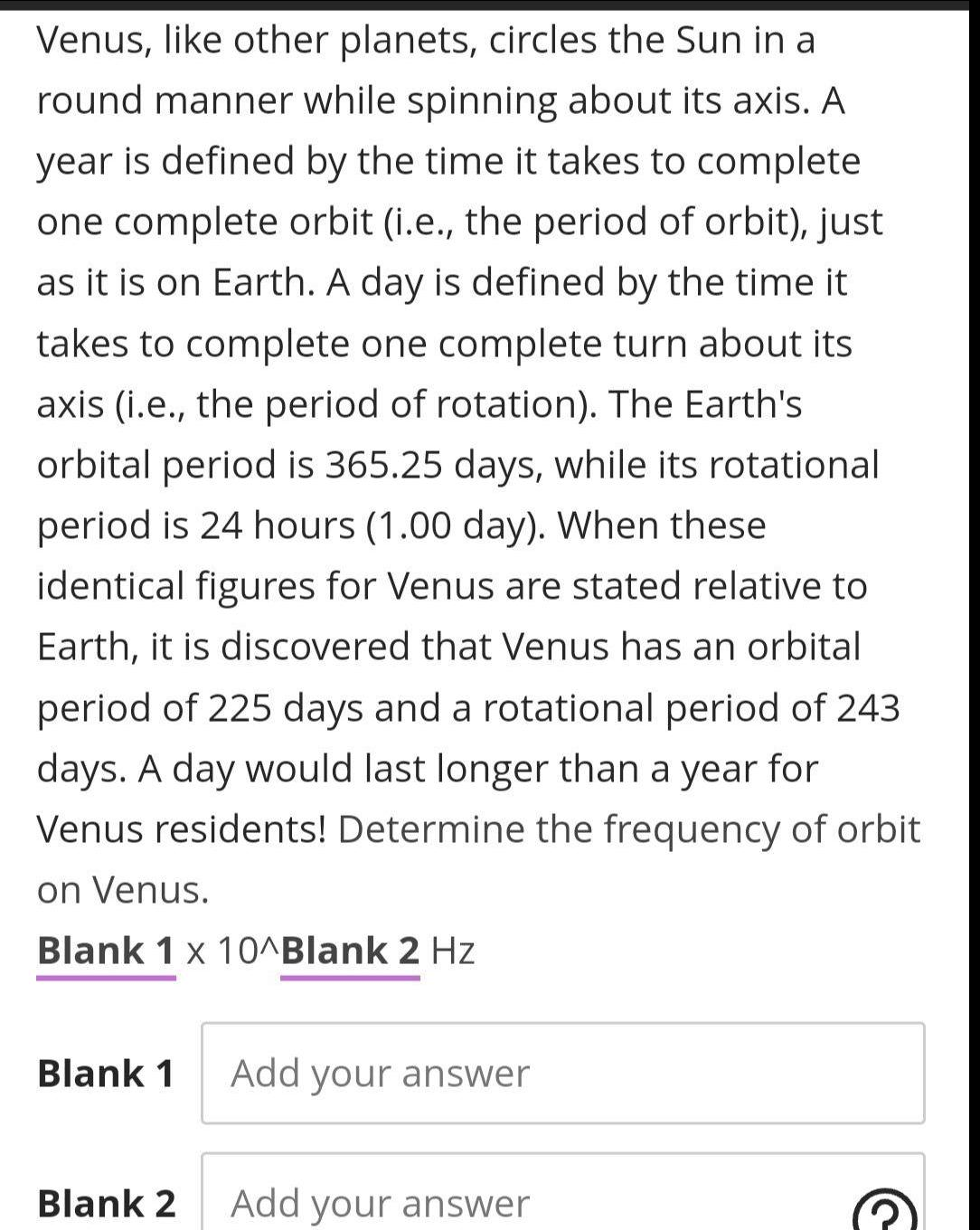Physics
Gravitation
Venus, like other planets, circles the Sun in a round manner while spinning about its axis. A year is defined by the time it takes to complete one complete orbit (i.e., the period of orbit), just as it is on Earth. A day is defined by the time it takes to complete one complete turn about its axis (i.e., the period of rotation). The Earth's orbital period is 365.25 days, while its rotational period is 24 hours (1.00 day). When these identical figures for Venus are stated relative to Earth, it is discovered that Venus has an orbital period of 225 days and a rotational period of 243 days. A day would last longer than a year for Venus residents! Determine the frequency of orbit on Venus. Blank 1 x 10^Blank 2 Hz Blank 1 Blank 2Physics
Gravitation
The gravitational field strength between two objects is the sum of two vectors pointing in opposite directions. Somewhere between the objects, the vectors will cancel, and the total force will be zero. Determine the location of zero force as a fraction of the distance r between the centres of two objects of mass m, and m₂.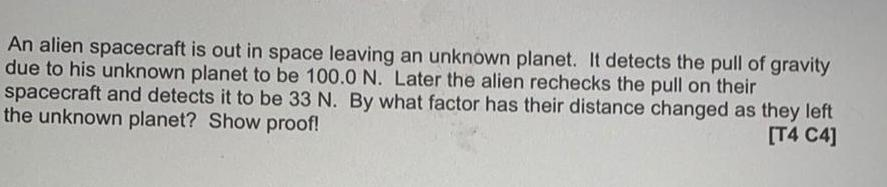Physics
Gravitation
An alien spacecraft is out in space leaving an unknown planet. It detects the pull of gravity due to his unknown planet to be 100.0 N. Later the alien rechecks the pull on their spacecraft and detects it to be 33 N. By what factor has their distance changed as they left the unknown planet? Show proof!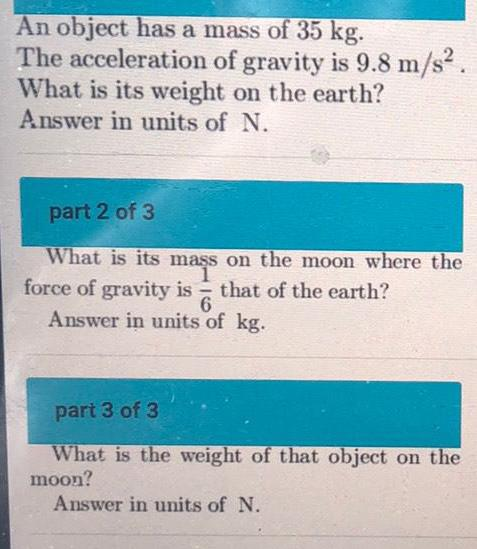Physics
Gravitation
An object has a mass of 35 kg. The acceleration of gravity is 9.8 m/s². What is its weight on the earth? Answer in units of N. What is its mass on the moon where the force of gravity is that of the earth? 6 Answer in units of kg. What is the weight of that object on the moon? Answer in units of N.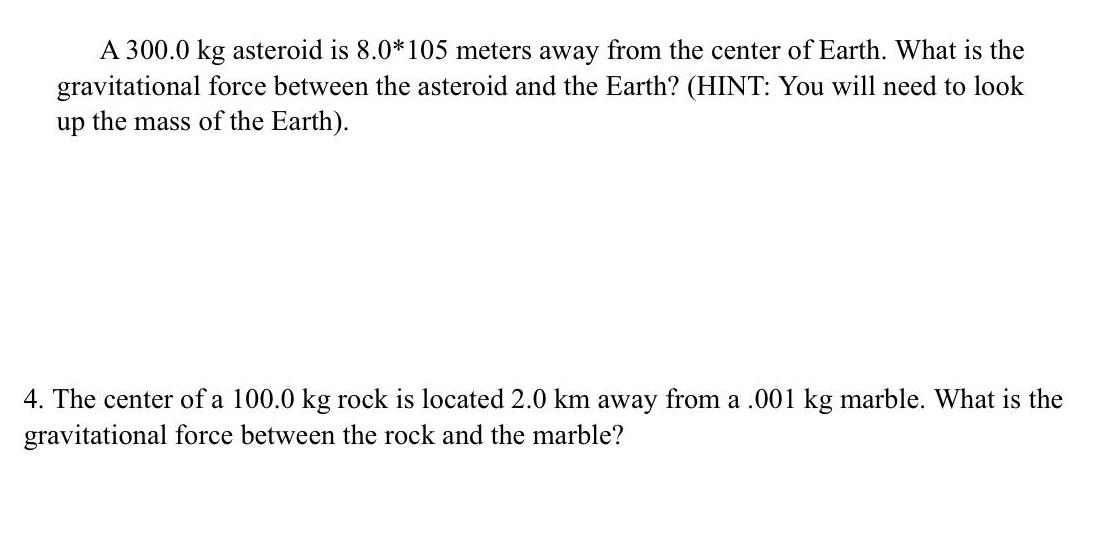Physics
Gravitation
A 300.0 kg asteroid is 8.0*105 meters away from the center of Earth. What is the gravitational force between the asteroid and the Earth? (HINT: You will need to look up the mass of the Earth). 4. The center of a 100.0 kg rock is located 2.0 km away from a .001 kg marble. What is the gravitational force between the rock and the marble?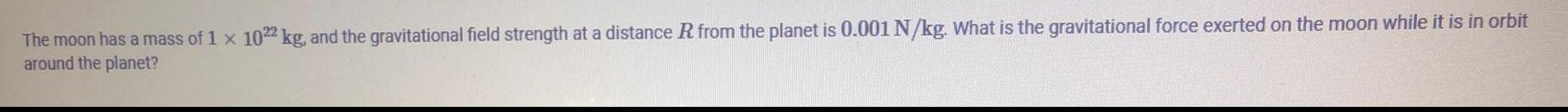Physics
Gravitation
The moon has a mass of 1 x 10^22 kg, and the gravitational field strength at a distance R from the planet is 0.001 N/kg. What is the gravitational force exerted on the moon while it is in orbit around the planet?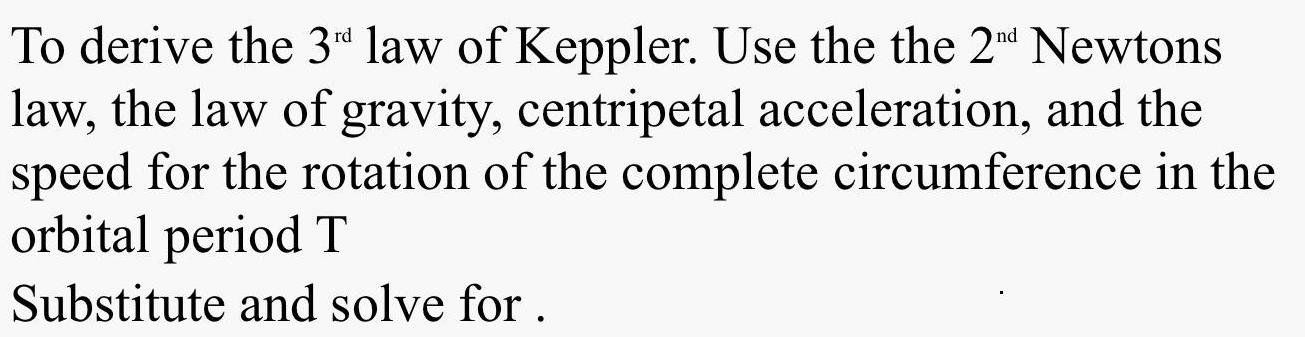Physics
Gravitation
To derive the 3rd law of Keppler. Use the the 2nd Newtons law, the law of gravity, centripetal acceleration, and the speed for the rotation of the complete circumference in the orbital period T Substitute and solve for.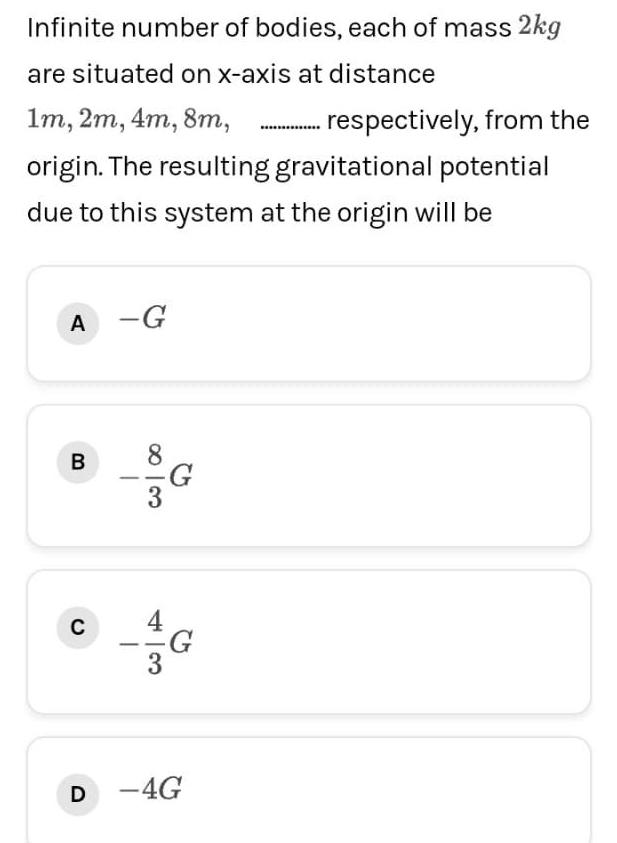Physics
Gravitation
Infinite number of bodies, each of mass 2kg are situated on x-axis at distance 1m, 2m, 4m, 8m, . respectively, from the origin. The resulting gravitational potential due to this system at the origin will be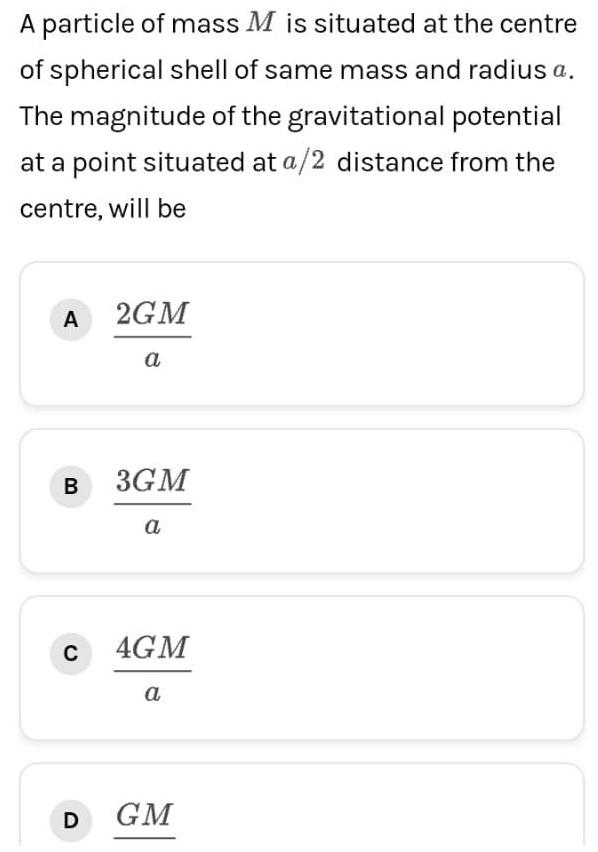Physics
Gravitation
A particle of mass M is situated at the centre of spherical shell of same mass and radius a. The magnitude of the gravitational potential at a point situated at a/2 distance from the centre, will be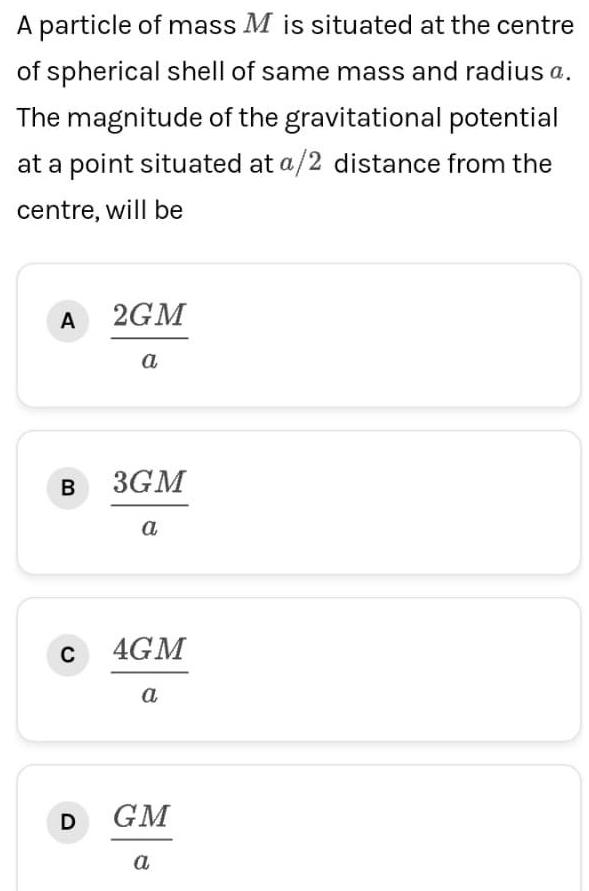Physics
Gravitation
A particle of mass M is situated at the centre of spherical shell of same mass and radius a. The magnitude of the gravitational potential at a point situated at a/2 distance from the centre, will be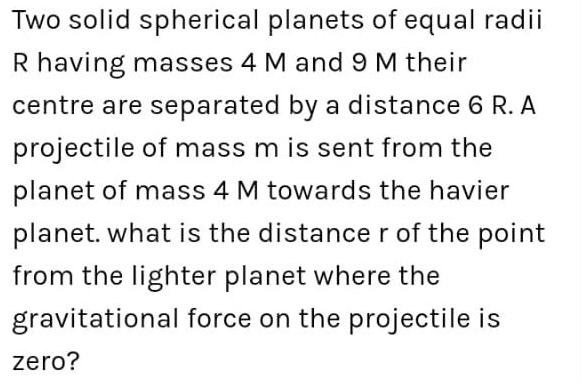Physics
Gravitation
Two solid spherical planets of equal radii R having masses 4 M and 9 M their centre are separated by a distance 6 R. A projectile of mass m is sent from the planet of mass 4 M towards the havier planet. what is the distance r of the point from the lighter planet where the gravitational force on the projectile is zero?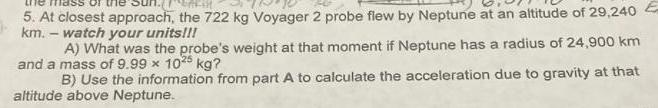Physics
Gravitation
At closest approach, the 722 kg Voyager 2 probe flew by Neptune at an altitude of 29,240 km. - watch your units!!! A) What was the probe's weight at that moment if Neptune has a radius of 24,900 km and a mass of 9.99 x 10²5 kg? B) Use the information from part A to calculate the acceleration due to gravity at that altitude above Neptune.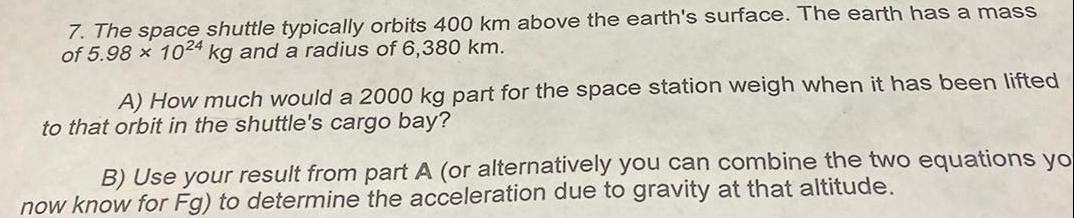Physics
Gravitation
The space shuttle typically orbits 400 km above the earth's surface. The earth has a mass of 5.98 x 1024 kg and a radius of 6,380 km. A) How much would a 2000 kg part for the space station weigh when it has been lifte to that orbit in the shuttle's cargo bay? B) Use your result from part A (or alternatively you can combine the two equations yo now know for Fg) to determine the acceleration due to gravity at that altitude.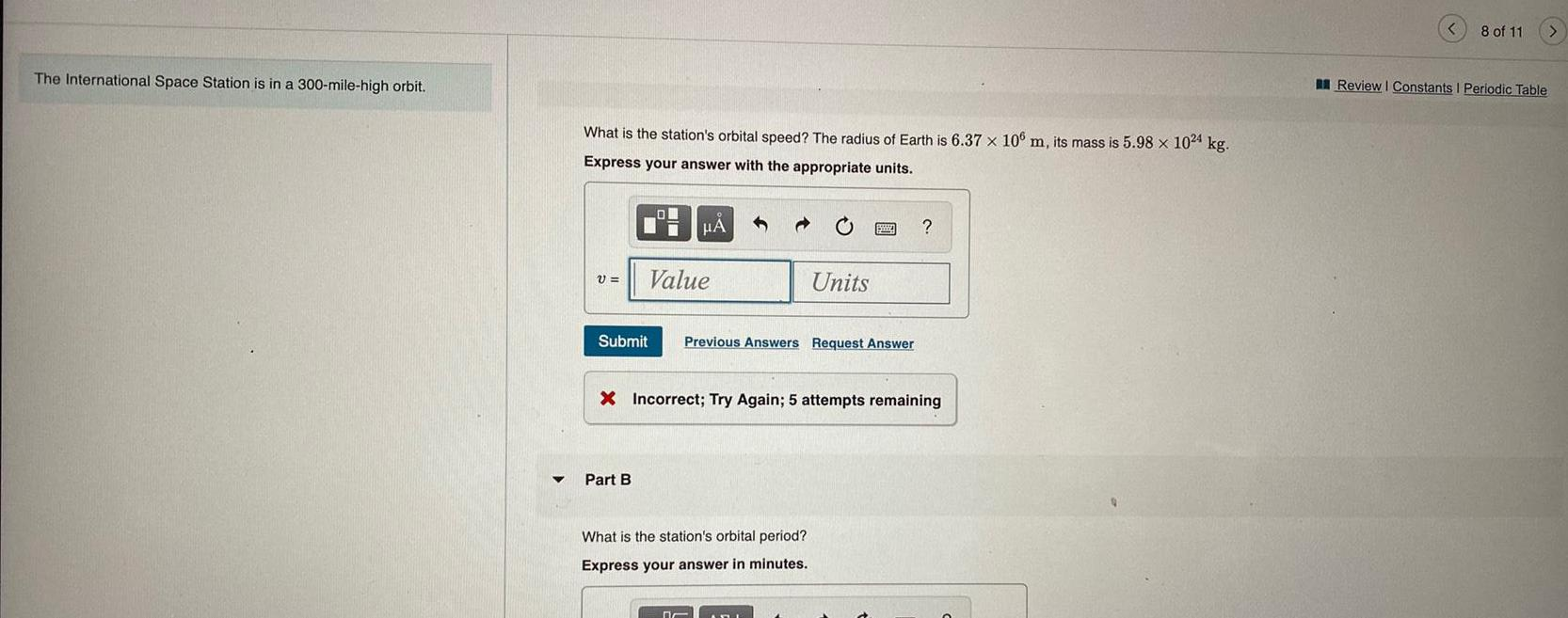Physics
Gravitation
The International Space Station is in a 300-mile-high orbit. What is the station's orbital speed? The radius of Earth is 6.37 x 106 m, its mass is 5.98 x 1024 kg. What is the station's orbital period?